# Glossary

## A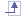activate This function is used to define a particular portion of a cloud for further operations.
align This function is used to reposition several clouds of points to each other in order to reconstruct a complete object.

## Ccloud of points A cloud of points is defined as a set of points in 3D space. It may consist of a single point or several million of points. Those points may be the result of a digitizing or of a computing operation.

In the current manual, the term cloud of points refer to several representations:

• representation as a set of points,
• representation as a set of lines of points (or scans),
• representation as a set of grids,
• representation as a mesh.
cell A cloud of points may consist of several cells (i.e. sub-clouds): for example, the cloud of points representing the handle below consists of two cells.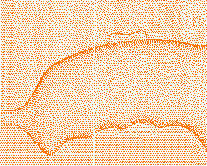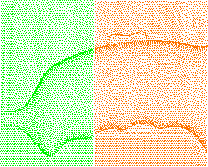characteristic line They are particular lines corresponding for instance to curvature variations (fillets start/end) or sharp edges, ...
cloud to align

In actions aligning clouds, the cloud to align is the cloud that is moved to be repositioned on another element.

constraint,
constraint element

In the action Align with constraints, setting a constraint consists in pairing two elements, one on the cloud to align, the second on the reference, to define the repositioning. Those two elements are called constraint element.

## Ffiltering Filtering a cloud of points is a method to create a lighter working context: some points are hidden, thus making further operations on the cloud quicker. Those hidden points can be recalled later.
flipping edge An edge common to two triangles of a polygon may be flipped, that is rotated, to respect the shape a sharp edge of the meshed part.

## Mmesh A mesh consists of a set of polygonal faces (triangles) which represent the surface of a 3D model. A triangulation is computed to describe the neighborhood relation of all points.

A mesh can be used to check the quality of the points, or can be processed in other applications.

A mesh may present some irregularities such as:

• Corrupted triangles, i.e. triangles that have the same vertex twice,
• Duplicated triangles, i.e. triangles that share the same three vertices,
• Non-manifold edges, i.e. edges shared by more than two triangles,
• Non-manifold vertices, i.e. vertices shared by two or more connected shells.

A mesh may also present some structural problems such as:

• Orientation problems, i.e. all the triangles are not oriented in the same direction,
• Isolated triangles, i.e. triangles belonging to small connected areas of the mesh,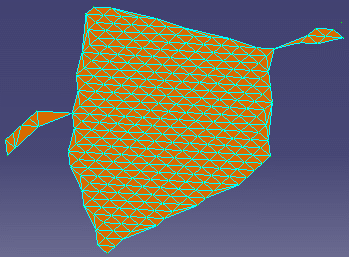• Disconnected zones, i.e. the mesh is made of several disconnected zones,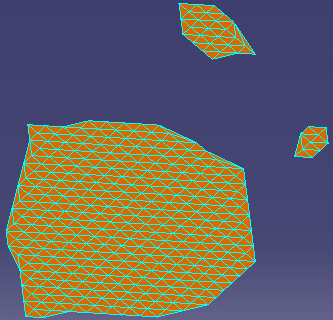• Triangles with long edges.

## Nneighborhood Many functions in Digitized Shape Editor operate on the points in space, regardless of the organization of the data in the cloud. In these functions, you can specify a maximum distance (neighborhood) which will be considered around a point for the operation. The larger the neighborhood value, the more points will be considered, and possibly the operation may become slower.

A default neighborhood value is proposed in those functions.

## Rreference In actions aligning clouds, the reference is the target, i.e. the element on which a cloud will be repositioned.
remove This function deletes physically points from the cloud of points. The points can not be recovered.
RPS Reference Point System.
It is defined by points such as centers of slots, cylinders, or other canonical geometrical shapes.
It defines a product (parts, its assembly or sub-assemblies) in a pre-established coordinate system.
RPS can be used to determine the alignment of the assembly tool such as assembly fixtures, and assembly line.
It can also be used to make quality control, by checking the tolerance between the nominal points and the actually measured points on physical parts
RPS constraints The constraint used in RPS alignment is called RPS constraint.
A RPS constraint is defined by a pair of points and/or circles respectively in the cloud to align and in the reference, plus the frozen axes.

## Sscan Cloud of points can be organized in consecutive scans, that is points in parallel planes.
sampling This operation is performed while importing a cloud of point. You can choose to import only a percentage of the points of the cloud.
shading A mesh can be visualized in shaded mode. This mode is a  method for visualizing the point data and getting an impression of its quality.

## Wworking distance This is the distance beyond which the elements are not taken into account for a computation.